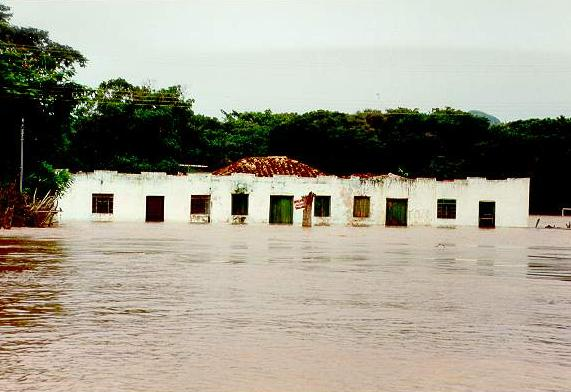online_dispersivity:  Calculation of convection, diffusion, and dispersion coefficients
of flood wave propagation[ SI Units:  uo in m/s; yo in m ]
[ U.S. Units:  uo in fps; yo in ft ]

Reference

F = uo / (g yo)1/2

V = (β - 1) F

qo = uo yo

Lo = yo / So

c = [ 1 + (V / F ) ] uo

ν = (Lo / 2) uo ( 1 - V 2 )

η = (Lo / 2) 2 uo ( 1 - V 2 ) F 2

c' = 1 + (V / F )

ν' = (1/2) ( 1 - V 2 )

η' = (1/4) ( 1 - V 2 ) F 2

F = Froude number;
V = Vedernikov number.

 INPUT DATA:

Select:

 SI units (metric) U.S. Customary units

Mean velocity uo :

Flow depth yo :

Channel slope So :

Rating exponent β :

 OUTPUT: SI units (metric) ♦  Froude F :    ♦  Vedernikov V :    ♦  Celerity c :      ♦  Diffusivity ν :      ♦  Dispersivity η :      ♦  Celerity c' :    ♦  Diffusivity ν' :    ♦  Dispersivity η' :

Press button to
or recalculate

Your request was processed at  01:45:43 am on May 30th, 2023   [ 230530  01:45:43 ].

Thank you for running   online_dispersivity   Please call again.